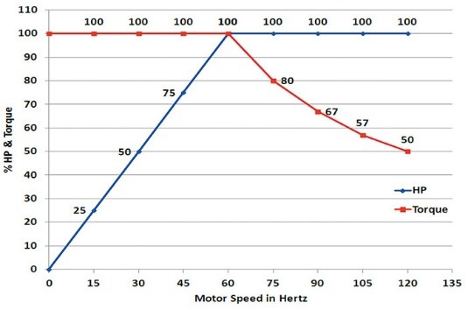• Home
• -
• Variable Frequency Drive: Torque vs. HP

# Variable Frequency Drive: Torque vs. HP

## HP & Torque Relationship

The relationship between the HP and torque produced by an electric motor can be a bit complicated. That relationship gets much more confusing when that motor is controlled by a Variable Frequency Drive (VFD). Learn the VFD need to knows.

In a linear situation, work is the product of the force applied to an object and the distance the object travels due to that force:

w = fd

In a rotational situation, torque is the equivalent of work. In this case the equation is slightly more complex. Torque is equal to the force applied, multiplied by distance from the axis of rotation (radius) and the angle (θ) at which the force is applied:

t = f(r sin θ)

Torque is measured in pound feet (lb-ft). Previously, it was foot pounds (ft-lbs), many still use this older term. Torque expresses how much work is performed, but it doesn’t indicate how quickly that work is completed.

Power is the rate at which torque (work) is performed:

p = t/time

Horsepower (HP) is the unit of power used in the US. The two equations below show the relationship of HP and torque when a motor’s speed changes. 5,252 is the constant, this is

the result of dividing James Watt’s original test data (33,000) by 2 pi (π).

HP = (t x rpm) / 5,252

t = (HP x 5,252) / rpm

According to the equation, torque must double if HP is to remain constant when speed is reduced by one half. Motors must do twice as much work per rotation to produce the same HP at the lower speed, this requires twice as much torque. That’s why the shaft and frame of a 900-rpm motor are usually larger than those of an 1,800-rpm motor of the same HP.

## How a VFD Affects Torque and Horsepower

HP or torque will change depending on the change in frequency when the speed of an AC motor is controlled by a VFD. Figure 1 below visually illustrates these changes. The X axis is motor speed from 0 to 120 hertz. The Y axis is the percent of HP and torque. HP and torque are both 100 percent at 60 hertz (base motor speed). When the VFD reduces frequency and motor speed, it also reduces voltage to keep the volts/hertz ratio constant. Torque remains at 100 percent, but HP is reduced in direct proportion to the change in speed.

At 30 hertz, the HP is 50% of the full HP at 60 hertz. The reason this occurs is because the total torque produced per unit of time is also reduced by 50 percent because of fewer motor rotations. The HP and torque equations can be used to verify this relationship.

##Figure 1. HP and torque changes with different frequencies

HP and torque flip situations when a VFD increases frequency above 60 hertz. , and torque decreases as frequency increases above 60 hertz, while HP remains at 100%. The torque reduction occurs because motor impedance increases with frequency increases. A VFD cannot increase the voltage above its supply voltage. This causes the current to decrease as frequency increases, thus decreasing the available torque.

Theoretically, torque is reduced by the ratio of the base speed divided by the higher speed

(60 hertz / 90 hertz = 67%). In real applications, other factors (increased bearing friction, increased fan loading, additional rotor windage, etc.) can reduce the actual available torque well below the theoretical values shown in Figure 1. At speeds above 60 hertz a motor’s full-load torque must be de-rated. Conventional manufacturers’ de-rating guidelines suggest using the base frequency to maximum frequency ratio for speeds up to 90 hertz. The square of the ratio is often used at speeds above 90 hertz.

End users should check with Kurz Industrial Solutions before running a motor above its base speed. Rotor balance, bearing life, and critical speed are typical areas to be affected. 1,800-rpm and 1,200-rpm motors up to 200 HP should be able to handle up to two times the base speed without problems. Overspeed is usually not at all recommended on 3,600-rpm motors over 50 HP. National Electrical Manufacturers Association (NEMA) MG1 gives overspeed guidelines that manufacturers must abide by.

A novel way to provide constant torque at higher-than-base speeds is to run a 230-volt motor on a 460-volt VFD. If you’ve done this you probably noticed a lot of smoke in the room. There are, however, applications in which this can actually work. In these applications, the drive is programmed to provide full voltage at 120 hertz and then reduce voltage proportionally as speed is reduced. The volts/hertz ratio remains at a constant 3.83 throughout the speed range. At 90 hertz the output voltage would be 345 volts and at 60 hertz it would be at the motor nameplate voltage (230 volts).